# The Monty Hall Problem

The Monty Hall problem is an interesting exercise in conditional probability. It focuses on a 1970’s American television show called Let’s Make a Deal hosted by television personality Monty Hall.

The game would end with a contestant being shown 3 doors. Behind one of those doors, there was a prize. Behind the other 2, a goat. Monty Hall would ask the contestant to pick a door. He would then open the one of the two remaining doors showing the contestant a goat. Now with 2 doors remaining, he would ask the contestant if they wanted to change their selection. The question was – should they?

Let’s look at it. In the beginning of the game, we have 3 closed doors. We know 1 of these 3 doors has a prize behind it, the other 2 do not. So the probability of picking the right door at this point is 1/3.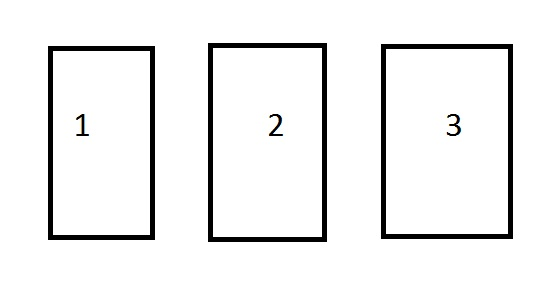Let’s say you chose door number 1. Once you chose, Monty Hall opens door number 3 and shows you the prize is not behind that door. He then asks if you would like to choose door number 2 instead.

So what do you think the probability the prize is behind door number 2? You have 2 doors left, so you might think it would 1/2. Well, you would be wrong.The reality is, the probability that the prize is behind door number 2 is actually 2/3.

Don’t believe me? Don’t worry, you aren’t alone. When Marilyn vos Savant wrote about this in her newspaper column, outraged mathematics professors from all over wrote her letters protesting her faulty logic. Her response was simple, play the game and see what results you come up with.

The trick to this neat little math problem is the unique constraints we are faced with. We start with 3 doors, from which we must pick 1. Giving us a the already agreed upon probability of 1/3.

Next, one of the two remaining options is removed the equations leaving only the door you have picked and 1 other door. Common sense would tell you that you now have a 50 – 50 situation where either door gives you the same odds of winning.

But common senses fails you. If you stick to your original choice, you will have a 1/3 probability of winning… but if you change your choice to the remaining door, your probability now shifts to 2/3.

Let’s see it in action.

Below is the layout of the three possible outcomes of the game.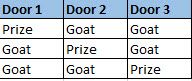For the sake of argument, let us say that we have decided to choose Door 1 to start the game.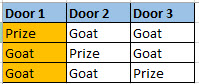After we have made that choice, one of the remaining doors with a goat behind it will be revealed.So, now we are left with the option to stick with door 1 or try the remaining door.

If we stick to our original door, we will win 1 out of the three possible games.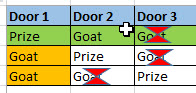If, however, you change your door after the reveal, you will win 2 times out of the 3 possible games.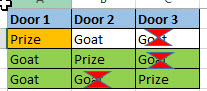## So what is the take away here?

If you ever find yourself on Let’s Make a Deal, always change your door after the reveal.

More importantly, if you are working with data for a living, make sure you have some healthy respect for the math behind statistics and probability. The Monty Hall Problem is, at its very heart, a conditional probability problem. Issues like this and Simpons’ Paradox can lead you to develop what appears to be logically sound models that are sure to fail once they come face to face with reality.

There is a lot of great software packages out there that make building machine learning models as simple as point and click. But without someone with some healthy skepticism as well as a firm handle on the math, many of these models are sure to fail.

# Bayes’ Theorem

Bayes’ Theorem sits at the heart of a few well known machine learning algorithms. So a fundamental understanding of the theorem is in order.

Let’s consider the following idea (the following stats are completely made up by the way). Imagine 5% of kids are dyslexic. Now imagine the tests administered for dyslexia at a local school is known to give a false positive 10% of the time. What is the probability a kid has dyslexia given the fact they tested positive?

What we want to know is = P(Dyslexic | Positive Test).

To figure this out, we are going to use Bayes’ TheoremDon’t worry. It is not all that complicated. Let’s break it down into parts:

• P(A) and P(B) are the probabilities of A or B happening independent of each other
• P(A|B) is the probability of A given the B has occurred
• P(B|A) is the probability of B given that A has occurred

Let’s take a new look at the formula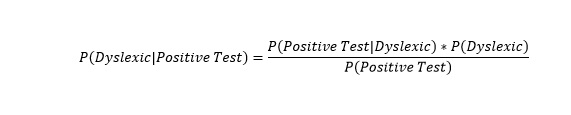So let me put this into English.

• P(Dyslexic|Positive Test) = probability the kid is dyslexic assuming he has positive test
• P(Dyslexic) = the probability the kid being dyslexic
• P(Positive Test) = Probability of a positive test
• P(Positive Test |Dyslexic) = The probability positive test assuming the kid is dyslexic

First, let’s figure out our probabilities. A tree chart is a great way to start.

Look at the chart below. It branches first between dyslexic and not dyslexic. Then each branch has positive and negative probabilities branching from there.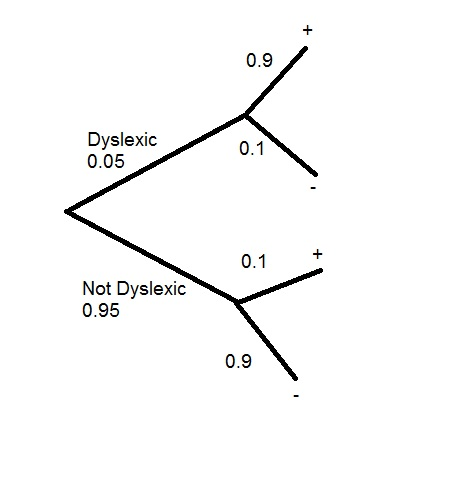Now to calculate the probabilities. We do this by multiplying the branches. For example Dyslexic and Positive  0.05 * 0.9 = 0.045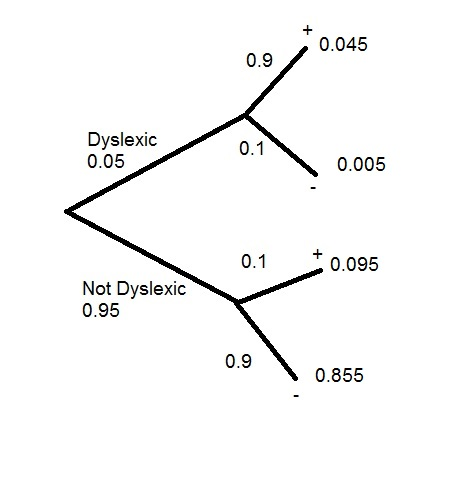Now, let’s fill in our formula. If you are having trouble seeing where the values come from look at the chart below

• P(Pos test | Dyslexic) = red * green = 0.05*0.9=.0.045
• P(Dyslexic) = First section of top branch = 0.05
• P(Positive Test) = red*green + yellow * yellow = 0.05*0.9+0.95*0.1=0.045+0.095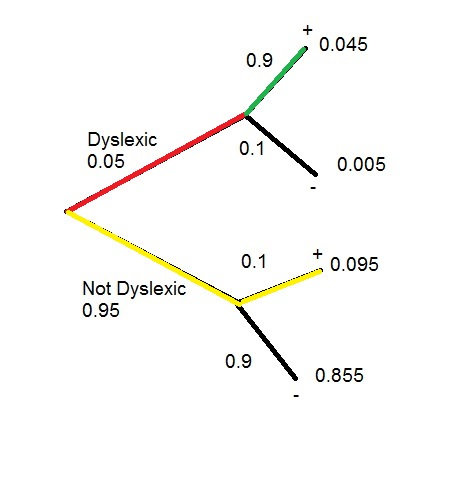So the probability of being dyslexic assuming the kid had a positive test = 0.016 or 1.6%

Another – perhaps more real world use for Bayes’ Theorem is the SPAM filter. Check it out below. See if you can figure your way through it on your own.• P(SPAM|Words) – probability an email is SPAM based on words found in the email
• P(SPAM) – probability of an email being SPAM in general
• P(Words) – probability of words appearing in email
• P(Words|SPAM) – probability of words being in an email if we know it is SPAM

# Probability: An Introduction

Many popular machine learning algorithms are based on probability. If you are a bit shaky on your probability, don’t worry this quick primer will get you up to speed.

Think about a coin flip. There are 2 possibilities you could have (heads or tails). So if you wanted to know the probability of getting heads in any particular flip, it would be 1/2 (desired outcome/all possible outcomes).

Now take a 6 sided die:

• The probability rolling a 1 is 1/6.
• rolling an even number (2,4,6) = 3/6 or 1/2
• rolling a number less than 3 (1,2) = 2/6 or 1/3

The compliment of a probability can also be referred to the probability of an event NOT happening. The probability of not rolling a 1 on a six sided die = 5/6.

P(~A) = 1 – P(A) = 1 – 1/6 = 5/6

## Independent Probability

Independent probability simply means determining the probability of 2 or more events when the outcome of one event has no effect on the other.

Let’s take two coins (A and B). Take the first coin and flip it. Imagine it comes up heads. Now flip the second coin. The fact that the first coin can up heads will not influence the outcome of the second flip in any way. To show this mathematically:

• Probability of flipping heads coin A = P(A) = 1/2
• Probability of flipping heads coin B = P(B) = 1/2
• Probability of flipping 2 heads = P(A and B) = P(A ∩ B) = P(A)*P(B)=1/2*1/2=1/4

## Mutually Exclusive

Now we are asking if event A or B occurred.

P(A or B) = P(A∪B) = P(A) +P(B)

So the probability of 10 or a 2 from a deck of cards = 1/52 + 1/52 = 2/52 = 1/26

## Not Mutually Exclusive

Imaging drawing an Ace and a Red Card. We want to make sure to factor in all the elements, but we need to account for double counting.

P(A or B) = P(A∪B) = P(A) +P(B) – P(A and B)

4/52 (ACE) + 26/52(Red Card) – 2/52(To get both an Ace and a Red card, the only options are Ace of Hearts and Ace of Diamonds) = 28/52 = 7/13

## Conditional Probability

Now we are going to work with dice. One six sided die and one 4 sided die. The diagram below shows all 24 possible combinations.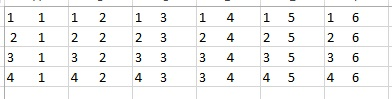Now conditional probability is the probability of something occurring assuming some prior event has occurred.  Look at the chart above, lets consider the A = rolling even number on six sided die (3/6) and B = rolling even number on 4 side die(2/4). So P(A|B) (read probability of A given B) = P(A∩B)/P(B). Lets look a the chart to help use see this.So, when rolling a six sided die (A), you have a 3/6 chance of rolling an even number(2,4,6)

When rolling a four sided die (B), you have a 2/4 chance of rolling an even number(2,4)

So P(A and B)  = 3/6*2/4=6/24=1/4

Now when figuring P(A|B) (rolling an even on the four side die assuming you have already rolled an even on the six sided die) we are no longer looking at all 24 combinations, we are now only looking at the combination where the six side die (A) is even (the green columns). So as you can see, of the 12 options where A is even, 6 have an even number on the 4 sided die.

So… P(A ∩ B)/P(B) = (1/4)/(1/2) = 1/2. Which makes sense since of the 12 combinations where A is even, 6 have even numbers for B. 6/12 = 1/2

# Probability vs Odds

Probability and odds are constantly being misused. Even in respected publications you will see sentences such as: “The odds of a Red Sox win tonight is 60%.” or “His probability is 2 to 1.” While in the lexicon of American English these words seem to have taken on interchanging meaning, when working with statisticians or data scientists, you are going to want to get your vocabulary straight.

## Probability:

Probability is a number between 0 and 1, often represented as a fraction or percentage. The probability is determined by dividing the number of positive outcomes by the total number of possible outcomes. So if you have 4 doors and 1 has a prize behind it, your probability of picking the right door is 1/4 or 0.25 or 25%.

Note, do not let the term positive outcome confuse you. It is not a qualifier of good vs bad or happy vs sad. It simply means the result is what you are looking for based on the framing of your question. If I were to state that out of every 6 patients who opt for this new surgery 2 die – 2 would be the “positive outcome” in my equation (2/6 or approx 33%) even though dying is far from a “positive outcome”.

## Odds:

Odds, on the other hand, are a ratio. The odds of rolling a 4 on a six sided die is 1:5 (read 1 in 5). The odds ratio works like this: positive outcomes : negative outcomes. So the odds of rolling an even number on a six sided die is 3:3 (or simplified to 1:1).

Now the probability of rolling an even number on a six sided die is 3/6 or 1/2. So keep that in mind, odds of 1:2 is actually a probability of 1/3 not 1/2.

### Deck of Cards:

Working with a standard deck of playing cards (52 cards).

Pulling a Red card from the deck

• probability: 26/52 = 1/2
• odds: 26:26 = 1:1

Pulling an Ace from the deck

• probability: 4/52 = 1/13
• odds: 4:48 = 1:12

Pulling a Diamond from the deck

• probability: 13/52 = 1/4
• odds: 13:39 = 1:3

# Python: Central Limit Theorem

The Central Limit Theorem is one of core principles of probability and statistics. So much so, that a good portion of inferential statistical testing is built around it. What the Central Limit Theorem states is that, given a data set – let’s say of 100 elements (See below) if I were to take a random sampling of 10 data points from this sample and take the average (arithmetic mean) of this sample and plot the result on a histogram, given enough samples my histogram would approach what is known as a normal bell curve.

In plain English

• Take a random sample from your data
• Take the average of your sample
• Plot your sample on a histogram
• Repeat 1000 times
• You will have what looks like a normal distribution bell curve when you are done.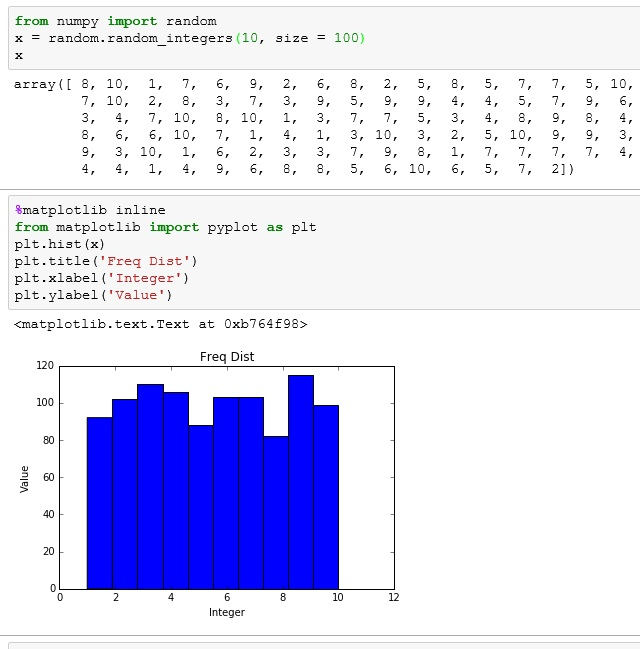For those who don’t know what a normal distribution bell  curve looks like, here is an example. I created it using numpy’s normal method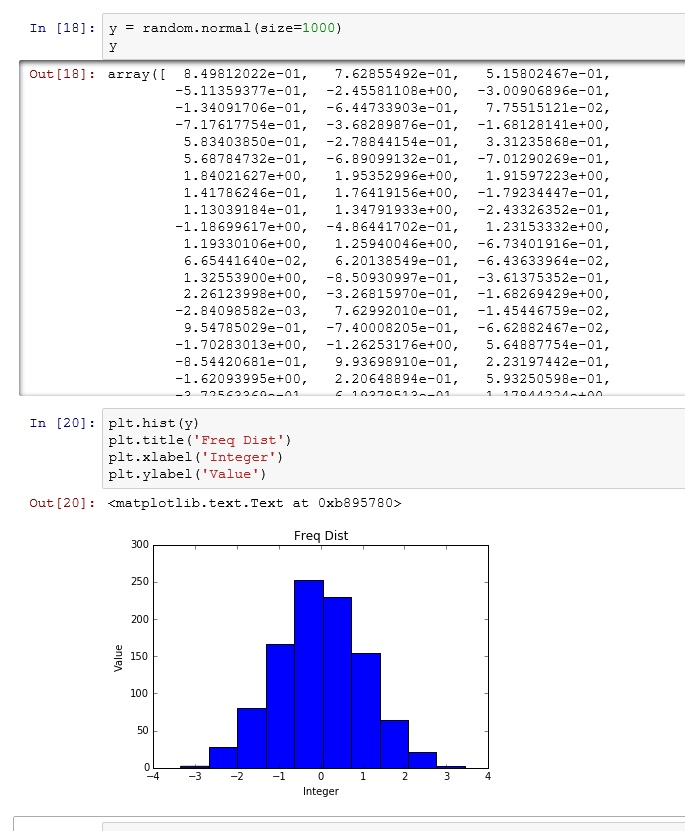If you don’t believe me, or want to see a more graphical demonstration – here is a link to a simulation that helps a lot of people to grasp this concept: link

## Okay, I have bell curve, who cares?

The normal distribution of (Gaussian Distribution – named after the mathematician Carl Gauss) is an amazing statistical tool. This is the powerhouse behind inferential statistics.

The Central Limit Theorem tells me (under certain circumstances), no matter what my population distribution looks like, if I take enough means of sample sets, my sample distribution will approach a normal bell curve.

Once I have a normal bell curve, I now know something very powerful.

Known as the 68,95,99 rule, I know that 68% of my sample is going to be within one standard deviation of the mean. 95% will be within 2 standard deviations and 99.7% within 3.So let’s apply this to something tangible. Let’s say I took random sampling of heights for adult men in the United States. I may get something like this (warning, this data is completely made up – do not even cite this graph as anything but bad art work)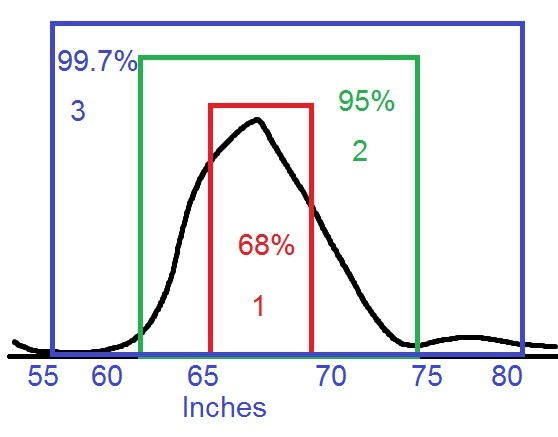But reading this graph, I can see that 68% of men are between 65 and 70 inches tall. While less than 0.15% of men are shorter than 55 inches or taller than 80 inches.

Now, there are plenty of resources online if you want to dig deeper into the math. However, if you just want to take my word for it and move forward, this is what you need to take away from this lesson:

## p value

As we move into statistical testing like Linear Regression, you will see that we are focus on a p value. And generally, we want to keep that p value under 0.5. The purple box below shows a p value of 0.5 – with 0.25 on either side of the curve. A finding with a p value that low basically states that there is only a 0.5% chance that the results of whatever test you are running are a result of random chance. In other words, your results are 99% repeatable and your test demonstrates statistical significance.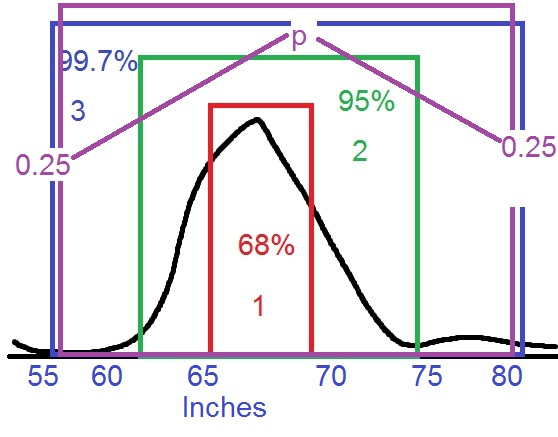# Python: Histograms and Frequency Distribution

In the spirit total transparency, this is a lesson is a stepping stone towards explaining the Central Limit Theorem. While I promise not to bog this website down with too much math, a basic understanding of this very important principle of probability is an absolute need.

## Frequency Distribution

To understand the Central Limit Theorem, first you need to be familiar with the concept of Frequency Distribution.

Let’s look at this Python code below. Here I am importing the module random from numpy. I then use the function random_integers from random. Here is the syntax:

random.random_integers(Max value, number of elements)

So random.random_integers(10, size =10) would produce a list of 10 numbers between 1 and 10.

Below I selected 20 numbers between 1 and 5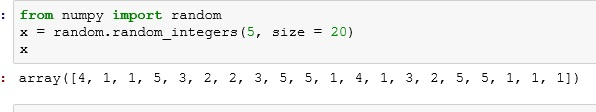Now, since I am talking about a Frequency Distribution, I’d bet you could infer that I am concerned with Frequency. And you would be right. Looking at the data above, this is what I have found.

I create a table of the integers 1 – 5 and I then count the number of time (frequency) each number appears in my list above.## Histogram

Using my Frequency table above, I can easily make a bar graph commonly known as a histogram. However, since this is a Python lesson as well as a Probability lesson, let’s use matplotlab to build this.

The syntax should be pretty self explanatory if you have viewed my earlier Python graphing lessons.Now lets, do it with even more data points (100 elements from 1 to 10 to be exact)If you enjoyed this lesson, click LIKE below, or even better, leave me a COMMENT.